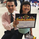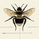5784 views
5784
There is a nice writeup about a system that uses DMISTO here, which includes decent statistics:

I have not yet done any back testing on the system as a whole myself, but thought the DMISTO was an interesting indicator, so ported it over for those who might want to play with it and create their own systems. I added dots that denote signals similar to the system described above, which can be turned off if desired.
study(title="TheLark: Directional Movement Index Stochastic", shorttitle="DMISTO_LK", overlay=false)

//•/•/•/•/•/•/•/•/•/•/•/•/•/•/•/•/•/•/•/•/•/•/•//
//                                             //
//              DMISTO BY THELARK              //
//                 ~ 8-4-14 ~                  //
//                                             //
//                     •/•                     //
//                                             //
//                                             //
//•/•/•/•/•/•/•/•/•/•/•/•/•/•/•/•/•/•/•/•/•/•/•//

// Wells Wilders MA
wwma(l,p) =>
wwma = (nz(wwma) * (l - 1) + p) / l

// Inputs
DMIlength = input(10,title="DMI Length")
Avglength = input(3, title="Avg Length")
ShowDots = input(true)
ob = input(90,title="Over Bought")
os = input(10,title="Over Sold")

// Osc Calc
hiDiff = high - high
loDiff = low - low
plusDM = (hiDiff > loDiff) and (hiDiff > 0) ? hiDiff : 0
minusDM = (loDiff > hiDiff) and (loDiff > 0) ? loDiff : 0
ATR = wwma(DMIlength, tr)
PlusDI = 100 * wwma(DMIlength,plusDM) / ATR
MinusDI = 100 * wwma(DMIlength,minusDM) / ATR
osc = PlusDI - MinusDI

// STO
hh = highest(osc,DMIlength)
ll = lowest(osc,DMIlength)
sto = 100 * (osc-ll) / (hh-ll)
kslow = sma(sto, Avglength)
perd = sma(kslow,Avglength)

// Plots
plot(ob,color=gray)
plot(os,color=gray)
plot(ShowDots ? kslow < perd and kslow > perd and perd < os ? os : na : na,style=circles,color=lime,linewidth=2)
plot(ShowDots ? kslow > perd and kslow < perd and perd > ob ? ob : na : na,style=circles,color=orange,linewidth=2)
plot(kslow, color=#0EAAEF,title="DMISTO-slow",linewidth=1)
plot(perd, color=red,title="DMISTO-slow",linewidth=1)
//plot(sto, color=#0EAAEF,title="DMISTO",linewidth=1)Super interesting, love the article! How does this differ from the stoch?